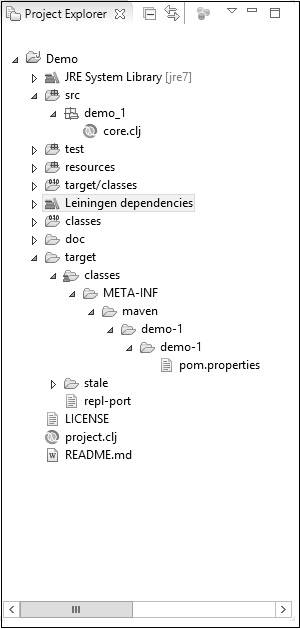### Clojure在线运行

`极速运行模式，更高效的运行，点击编辑器上方的运行按钮即刻体验吧。`

# 基本语法

## Hello World作为一个完整的程序

### 例

``````(ns clojure.examples.hello
(:gen-class))
(defn hello-world []
(println "Hello World"))
(hello-world)
``````

• 程序将被写入一个名为main.clj的文件中。 扩展名'clj'是clojure代码文件的扩展名。 在上面的示例中，文件的名称称为main.clj。

• 'defn'关键字用于定义一个函数。 我们将在另一章详细介绍功能。 但是现在，知道我们正在创建一个名为helloworld的函数，它将拥有我们的主要Clojure代码。

• 在我们的Clojure代码中，我们使用'println'语句将“Hello World”打印到控制台输出。

• 然后我们调用hello-world函数，它又运行'println'语句。

``````Hello World
``````

## 声明的一般形式

``````(+ 1 2)
``````

``````(str "Hello" "World")
``````

### 例

``````(ns clojure.examples.hello
(:gen-class))
(defn Example []
(println (str "Hello World"))
(println (+ 1 2)))
(Example)
``````

``````Hello World
3
``````

## 命名空间

### 语法

``````*ns*
``````

### 例

``````*ns*
``````

### 输出

``````clojure.examples.hello

(ns clojure.examples.hello
(:gen-class))
(defn Example []
(println (str "Hello World"))
(println (+ 1 2)))
(Example)
``````

## 加载Clojure包

Clojure代码封装在库中。 每个Clojure库都属于一个命名空间，类似于Java包。 您可以使用'Require'语句加载Clojure库。

### 语法

``````(require quoted-namespace-symbol)
``````

### 例

``````(ns clojure.examples.hello
(:gen-class))
(require ‘clojure.java.io’)
(defn Example []
(.exists (file "Example.txt")))
(Example)
``````

## Clojure中的注释

### 例

``````(ns clojure.examples.hello
(:gen-class))

;; This program displays Hello World
(defn Example []
(println "Hello World"))
(Example)
``````

## 分隔符

### 例

``````(ns clojure.examples.hello
(:gen-class))

;; This program displays Hello World
(defn Example []
(println (+ 1 2 3)))
(Example)
``````

### 输出

``````6
``````

### 例

``````(ns clojure.examples.hello
(:gen-class))

;; This program displays Hello World
(defn Example []
(println [+ 1 2 3]))
(Example)
``````

### 输出

``````[#object[clojure.core\$_PLUS_ 0x10f163b "clojure.core\$_PLUS_@10f163b"] 1 2 3]
``````

## 空格

```(+ 1 2 3 4 5) (+ 1, 2, 3, 4, 5)```

## 符号

``````tutorial-point!
TUTORIAL
+tutorial+
``````

## Clojure的项目结构• demo_1 - 这是Clojure代码文件放在其中的包。

• core.clj - 这是主要的Clojure代码文件，它将包含Clojure应用程序的代码。

• Leiningen文件夹包含像运行任何基于Clojure的应用程序所需的clojure-1.6.0.jar文件。

• pom.properties文件将包含诸如groupId，artifactId和Clojure项目版本等信息。

• project.clj文件包含关于Clojure应用程序本身的信息。 以下是项目文件内容的示例。

``````(defproject demo-1 "0.1.0-SNAPSHOT"
:description "FIXME: write description"
:url "http://example.com/FIXME"
:url "http://www.eclipse.org/legal/epl-v10.html"
}
:dependencies [[org.clojure/clojure "1.6.0"]])
``````

JSRUN提供的Clojure 在线运行，Clojure 在线运行工具，基于linux操作系统环境提供线上编译和线上运行，具有运行快速，运行结果与常用开发、生产环境保持一致的特点。

• 00:23